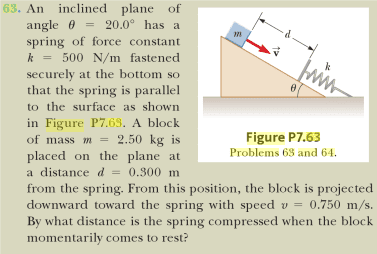# Systems of Energy & Work

FeelTheFire
Hey all, I was feeling confident with the idea of work when dealing with vectors and using the dot product to find the work.
However, recently we have begun to discuss work while considering the object experiencing the force as part of a system. I'm having trouble transitioning into this idea and I don't really know when to consider energies and apply the work energy theorem versus using vectors and dot products. An example that I'm having trouble with:The first thing I'm tempted to do is draw a free body diagram and begin plugging vectors into newton's second law.

I was able to solve the problem using a hint from my instructor but I don't understand why the method works. It was as follows:

Ki + Wg + Ws = Kf Where Ki is initial kinetic energy, Wg is the work done by the gravitational force, Ws is the work done by the spring force and Kf is the final kinetic energy. When you substitute and simplify all this down you can solve for the displacement (x) via a quadratic equation and arrive at 0.131 m.

We have not learned about the conservation of energy yet. Any insight would be much appreciated. I really want to understand this concept!

Last edited:

Homework Helper
Gold Member
Well that is odd that you would be given a problem that uses work-energy principles when you haven't been taught it yet. Your instructor has hinted at using the Work-Energy principle that states that the net or total work done on an object is equal to its change in kinetic energy. You could also use conservation of energy that states that the sum of the initial potential and kinetic energy is equal to the sum of the final potential and kinetic energy (in the absence of any work done by non conservative forces), which I thiink is a bit simpler because of issues with plus or minus signs. It isn't very helpful to use an equation you are not familiar with and just plug in numbers without knowing when or how to properly use the equation with little understanding of the theory behind it.

FeelTheFire
Thanks for the reply PhanthomJay, I appreciate it.

Well that is odd that you would be given a problem that uses work-energy principles when you haven't been taught it yet.

Sorry I must not have been clear. I have heard the lecture and read the text on the ideas of energy and work, but I'm having a hard time understanding and applying the new concepts.

In particular, I can't distinguish which problems would be better solved using energy concepts instead of Newtonian vector concepts. I don't really understand the difference or the NEED to work with energies.

Also, until you said it, I didn't even recognize the instructors hint as the work-energy theorem. I have never seen it used with multiple sources of work before, only as W = delta K.

The next chapter in my text deals with the "conservation of energy", and I'd really like to understand conceptually the idea of energies before I move on to it. So I guess my query is more about the nature of energy concepts rather than a specific problem...